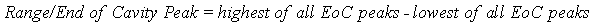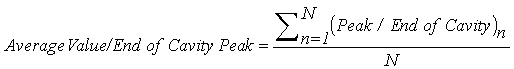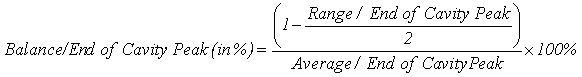Select Page

I have had some recent requests to distribute some of the formulas that the eDART™ uses to calculate summary values and some statistics. This week several have come in regarding the summary typeBalance”.

Note: In the following formulas the slash ( / ) separates type and location per the standard syntax used in these tips. It does not mean divide.

At the end of each shot (integration limit or end of screw run) the eDART first calculates all of the basic values that make up balance. In this example, after a Peak / End of Cavity value has been computed for each End of Cavity (“EoC”) sensor, the eDART calculates a single summary value “Range” thus:At the same time it creates an average of the peaks using the standard formula for mean (average):ThenThe division by 2 is to think in terms of the worst case spread either side of center.

What do you do with the Balance number?

Commonly customers set a warning, often non-sorting, on Balance or Range. This allows them to catch a spread in quality between cavities before it gets out of control. Balance / Cavity Fill Time may also be placed on the Cycle Values as a target when adjusting tip temperatures. And you may plot a trend on the Summary Graph for balance. This may show an oscillation in the hot runner controller drive to the tips

Note that the eDART computes balance only for cavity fill time (from Process Time / Cavity Fill) peak unless you install the Balance Viewer. When the Balance Viewer is running it computes balance for the remaining summary values: cooling rate, cycle and injection integrals, time-to-temperature sensor, temperature sensor minimum etc.